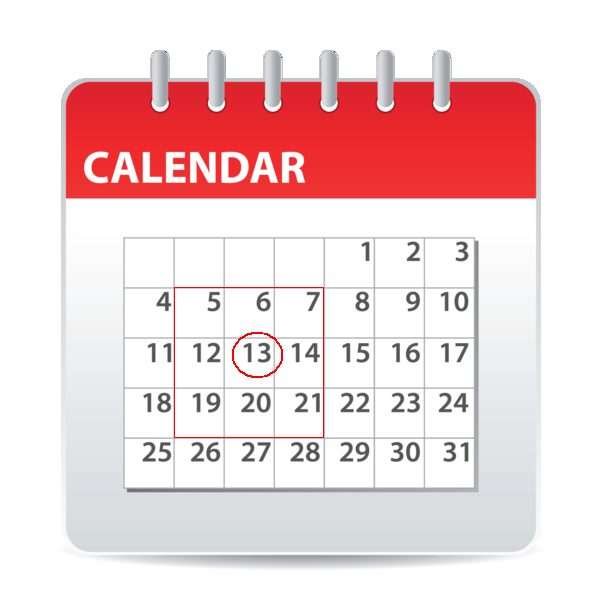Learn and Educate Yourself

## Calendar Magic – A Magical Math Puzzle

A simple Maths puzzle displaying Calendar Magic for the smart minds. Surprise anyone at home, school, in the college with this simple trick. A Math multiplication magic trick unknown to many of us. Impress Your friends with this hidden magical factor.

Guess the sum of 9 numbers in any 3 x 3 box of a Calendar?

Ask your friend to put a square around 9 numbers on a calendar in a box of 3 x 3. Now ask them to find the sum of 9 numbers within the square boxes of a calendar in a flash.### Calendar Magic Puzzle Solution

All we need to do is multiply the number in the center of the square by 9. That’s it.

Example:    13 x 9 = 117     Or    5 + 6 + 7 + 12 + 13 + 14 + 19 + 20 + 21 = 117

Find out more Maths Puzzles